# Harsh Bhatia

Lawrence Livermore National Laboratory, P.O. Box 808, L-478, Livermore, CA - 94551

### Simplifying the Analysis of Unsteady FlowsSee details

### Obtaining Consistency in Data Analysis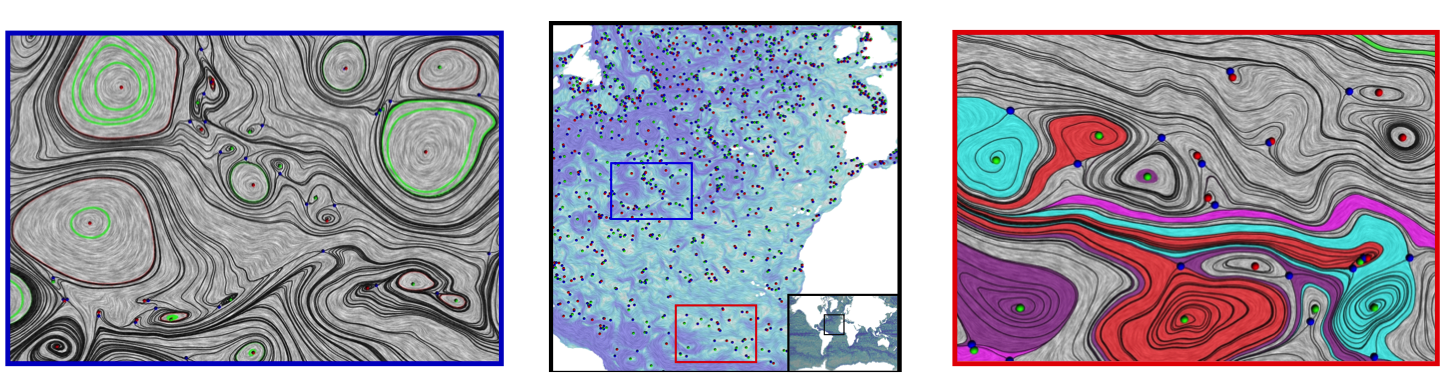See details

### Eliminating the Boundary Artifacts from the HHD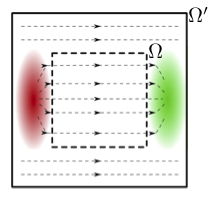See details

### Simplifying Jacobi Set for Multi-Scalar-Field Analysis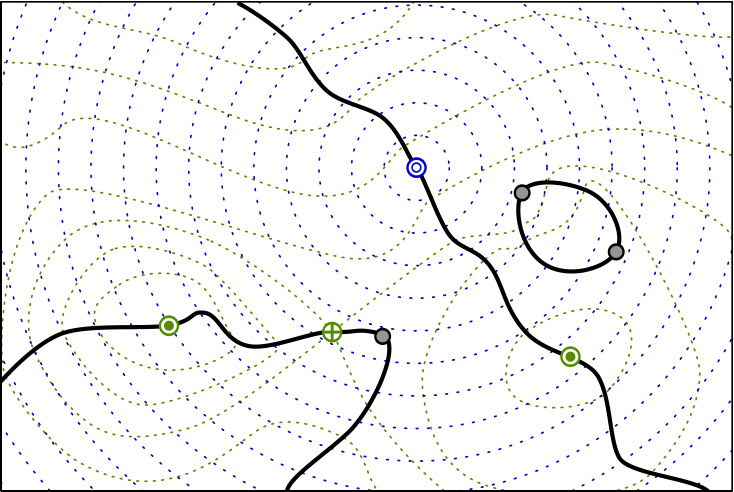See details

### Visualizing Robustness of Critical Points in 2D Vector Fields### Mobility Analysis in Wireless Sensor Networks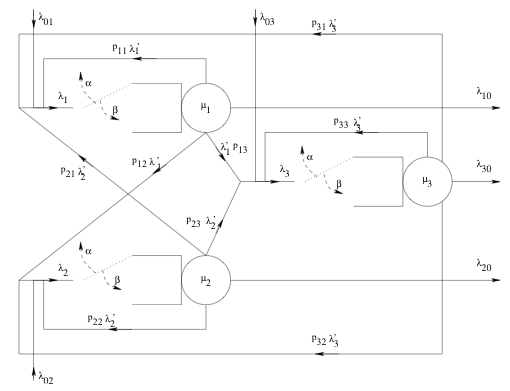### Simplifying the Analysis of Unsteady Flows

The inclusion of temporal dimension in data greatly increases the complexity of analysis, especially in the case of flows, i.e., unsteady (time-varying) flows. The current techniques for analyzing such flows are computationally prohibitive, and typically, cause data management issues by requiring simultaneous access to a large amount of data. For example, the pathline-based techniques have high computational cost, and typically use numerical integration which is prone to errors. These techniques must also require the data for a finite amount of data for pathline-integration.

The focus of this work is to simplify the analysis of unsteady flows by means of new mathematical models and robust computations allowing for lower cost and better data management. In particular, we are working on finding new reference frames which can enable the much-simpler analysis techniques for steady flows for the unsteady case.

### Eliminating the Boundary Artifacts from the Helmholtz-Hodge Decomposition

The Helmholtz-Hodge decomposition (HHD) is a fundamental theorem in fluid dynamics, describing a vector field as a sum of a divergence-free, a curl-free, and a harmonic vector field. The HHD, however, describes an underspecified system on domains with boundary, and therefore, is not unique. The traditional way to obtain uniqueness is to impose boundary conditions. For example, the most common boundary conditions demand that the divergence-free and curl-free components must be normal and parallel to the boundary. While these boundary conditions work well for certain cases, imposing a standard set of boundary conditions irrespective of the data can produce aribtrary artifacts. Although the issue of boundary conditions has been acknowledged by many, currently, there exists no better solution to obtain uniqueness.

From the potential function theory, we know that harmonic functions are always created due to external influences with respect to the domain. Using these concepts, we have developed a new technique to obtain uniqueness, where one does not need to assume any boundary conditions a priori. In contrast, the decomposition naturally determines the flow on the boundary.Potential function theory tells us that harmonic functions are always created due to external influences with respect to the domain. For example, the linear flow in the smaller domain is harmonic, and is created due to positive and negative divergence (in red and green) outside.

### Obtaining Consistency in Data Analysis

Computational tools for data analysis are based on mathematical foundations that assume smooth functions defined over smooth domains, and require the availibility of infinite-precision real numbers to represent the data. However, in practice, these computational framworks are limited to finite-precision floating point numbers, and can only represent interpolated functions over sampled domains. As a result, the computational realization of the underlying mathematical theory suffers from unavoidable numerical errors.

Understanding, capturing, and visualizing these errors during the analysis and visualization pipeline of scientific data is extremely important, especially because such errors can cause the results to become inconsistent with the fundamental theory, and therefore, the analysis may not be physically meaningful. For example, in an experiment measuring the gravitational acceleration, we may get the result to be 10, or 9.8, or 9.807, depending upon the accuracy of the system. While it is important to quantify this error, an even more serious problem appears if the system shows direction of this acceleration to be upward - clearly, a physically incorrect result. In the context of analysis, we call this result to be "inconsistent" with the theory.

The focus of this project is the development of new computational tools that provide consistency guarantees during analysis. The goal is to eliminate most numerical errors through more sophisticated representations of the data, and to capture the unavoidable errors to visualize them as well as to enforce consistent results. The achieved consistency guarantees lead to a greater confidence in analysis results when they are used for deriving scientific insights.

#### Consistent Streamline Tracing in Sampled Vector Fields

Streamlines are curves that are everywhere tangent to the direction of vector field. The theory of vector fields (and dynamical systems) dictate that streamlines must be mutually non-intersecting, meaning two distinct streamlines must not cross. However, since streamlines are most commonly computed using numerical integration, the associated errors get compounded and can become unbounded, possibly resulting in intersecting streamlines. Such analysis is scientifically misleading and must be avoided.

In a series of papers, we developed a new representation of sampeld vector fields, which we call the "Edge Maps", to overcome these inconsistency issues. The Edge Maps represent the streamlines as they travel from edge to edge in a triangulated domain using a highly accurate streamline tracer, thus bypassing most of the numerical errors in an offline computation. In the runtime, the system can use Edge Maps to trace streamlines interactively with an additional benefit of visualizing the unavoidable errors. Most importantly, it becomes possible to extend the Edge Maps to a combinatorial representation by finely discretizing the triangles' edges. This allows to replaced floating-point arithmetic with integer arithmetic, enabling the system to enforce consistency.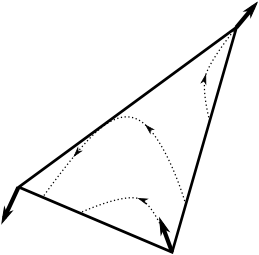Left to right: The traditional way of storing vector fields as samples on the vertices. Capturing the behavior of streamlines by partitioning the boundary of the triangle. Constructing the Edge Maps using these boundary partitioning. Discretizing the boundary gives a discrete representation that can be used for consistent streamline tracing.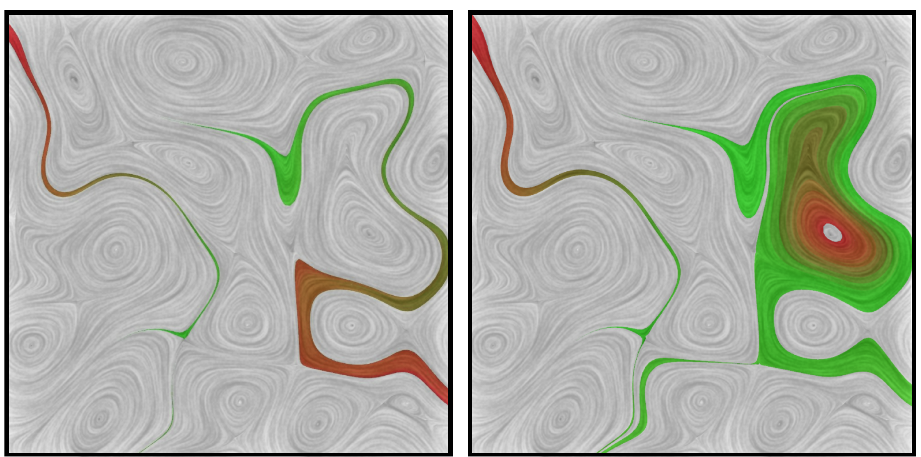Visualizing spatial and temporal error in streamline tracing using Edge Maps. Left and middle: Possible locations where a point may travel to under the given amount of error. Right: Possible locations where a point may exist at a given time under a given amount of error.

#### Consistent Singularity Detection in Sampled Vector Fields

Detection of singularities is one of the most important aspects of analysis, since they represent important features in the data. In the case of vector fields, the singularities must follow certain rules with respect to their spatial distribution, for example, between two sources (that create the flow), there must be a sink (that destroys the flow) or a saddle (that reroutes the flow). Formally, these laws can be summarized using the Poincare-Hopf index formula. The use of numerical techniques to detect singularities can, however, violate these laws, resulting in physically meaningless results.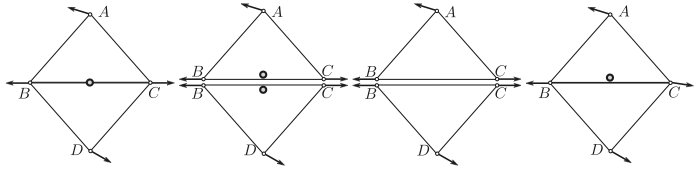In an unstable configuration as shown (leftmost), where a singularity lies on an edge shared by two triangles, common numerical techniques may detect false positives (second from left) or false negatives (third from left), which are both inconsistent configurations. A consistent singularity detection (rightmost) must consistently choose a single triangle to represent the singularity.

To address these issues, we developed mathematical conditions required for the existence of a singularity in a simplex (a triangle in 2D, or a tetrahedron in 3D). Using a combinatorial representation and integer arithmetic, we develped a computational framework that consistenty detects the singularities in a vector field.